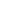User Rating: 3 / 5The RSI is a momentum metric that helps to determine when it is a good moment to sell or buy. It's valued with nondimensional units between 0 and 100. The basic formula to calculate it is given by:

`RSI(t) = 100 - 100 / (1 + RS(t))`
`RS(t) = Average gain of up periods during the specified t time frame / Average loss of down periods during the specified t time frame`

Where:

• t is the number of periods to evaluate, usually, 14 is a good value

The interesting thing on this metric is that it will work regardless the range of the variation. Thanks to this metric, you can see the likelihood of a given price. But, you can use it to know when to trade. Usually, when RSI returns a value higher than 70 it means it is a good moment to sell and lower than 30 it would be a good moment to buy.

With a little more of statistics, this metric could be used determine if a currency is a candidate for trading. For example, if a pair of a given time period hits many times the low and high thresholds, it could indicate the currency has enough fluctuations for trading. Of course, more conditions must meet.

Good luck!